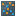#### Versions

You are viewing the RapidMiner Studio documentation for version 8.2 - Check here for latest version

#Lift Chart (Simple) (Model Simulator)

## Synopsis

This operator creates a lift chart which shows how much better the model performs for each confidence segment than random guessing.

## Description

A lift chart shows how much better a machine learning model performs compared with a random guess. It also shows you the point at which the predictions become less useful. This is in particular useful if you can optimize for a cost-benefit ration as it often happens for marketing-related use cases. For example, a lift chart can show you that to reach 80% of your respondents you only need to reach out to 30% of your total address base.

The lift chart shows you 10 bins for your test data. Each bin is filled with decreasing confidence of the model for the target class. That means that the examples with highest confidence values are in the first bin, then in the second, and so on. The chart consists of two parts. The bars show you the correct percentage for the target class. For example, if the first bar in the lift chart shows 95%, this means that 95% of all examples in this confidence bin are actually from the desired target class.

The second part of the chart is a line which shows you the cumulative coverage of the target class if you would consider only examples of at least the confidence of the corresponding bar or higher. A value of 60% at the third bar for example means that you covered 60% of the desired target class at that point. But the third bar only represents 30% of your total population. That means that this model would correctly identify 60% of the target with only using 30% of the total population (the 30% with the highest confidence for this class). In contrast to this, a random model would only achieve 30% of the target class.

## Input

•model (Centroid Cluster Model)

This input port expects a prediction model.

•test data (Data Table)

The test data to create the lift chart for. Needs a label attribute to compare with model predictions.

## Output

•lift chart

The lift chart for the given test data.

## Tutorial Processes

### Lift Chart for Naive Bayes on Titanic

This process creates a model on the Titanic data set. It first divides the data into a training and testing part. The model is built on the training data. It is then delivered together with the test data to the Lift Chart operator. Please note that you need to specify which class you are interested in. You can do this in the parameters of this operator.

Examining the lift chart, we can see that you can correctly identify 47% of all survivors while you are only looking at the first 20% of the passengers.